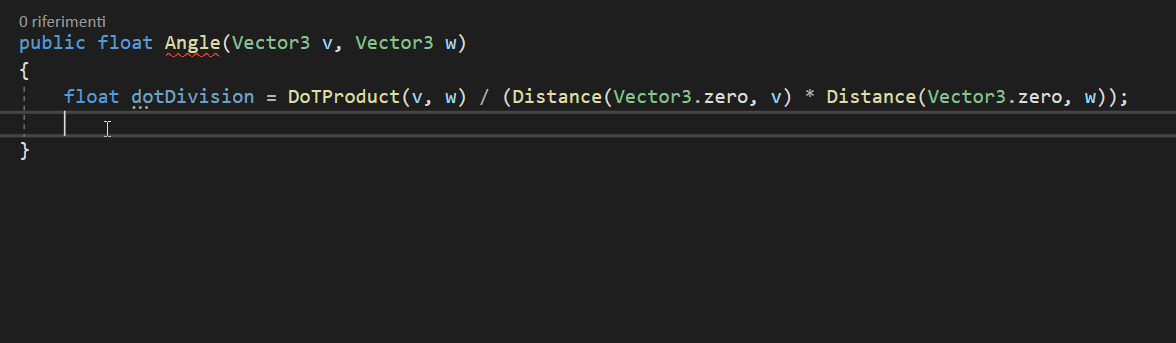# we will take the x of v and we will multiply it by the x of w, same thing with the y, so vy * wy, and we will add them.

## Small note: obviously these formulas work in both 2D and 3D, the only difference is that the z axis should be added to the calculation.((v.x * w.x) + (v.y * w.y) + (v.z * w.z));

To make less confusion, however, I will change the parameter from Vector2 to Vector3.

# Angle = inverse cosine of the DotProduct divided by the length of the Vector v multiplied by the length of the Vector w.

It always seems complicated, but it is actually much more “understandable” written this way.
We now have the DotProduct formula.
And also the “Length” formula because the Distance () method returns exactly the Length of a Vector.

# Next we divide by the length of v and w multiplied by each other.Acos() needs a float and we can pass dotDivision as a parameter.

--

--## Matteo Lo Piccolo

Always in love with programming, even if late (I'm already 39 years old) I decided to follow my dream! We will see how far my passion will take me!